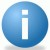# Sigma to DPMO to Yield to Cpk Table

ByiSixSigma-Editorial
 Sigma DPMO Yield Cpk 1.5 500,000 50% 0.50 3.00 66,800 93.320% 1.00 3.50 22,700 97.730% 1.17 4.00 6,210 99.3790% 1.33 4.50 1,350 99.8650% 1.50 5.00 230 99.9770% 1.67 6.00 3.4 99.99966% 2.00

Assumptions
No analysis would be complete without properly noting the assumptions made. In the above table, we have assumed that the standard sigma shift of 1.5 is appropriate (the calculator allows you to specify another value), the data is normally distributed, and the process is stable. In addition, the calculations are made with using one-tail values of the normal distribution.

Handpicked Content:   Understanding Process Sigma Level

Discussion
If you are starting with DPMO, convert it to a decimal value by dividing by 1,000,000. If you multiply that decimal by 100, that is your Yield (%). If you take the decimal and look it up in a standard normal curve (Z table), you can determine the corresponding Z which is the long term Z. To convert to short term Z, which is the Sigma level, use the following formula:

Z(short term) = Z(long term) + 1.5

Then you can determine Cpk using the following formula:

Cpk = Z(short-term) / 3